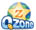## 1546: Just Pour the WaterShirly is a very clever girl. Now she has two containers (A and B), each with some water. Every minute, she pours half of the water in A into B, and simultaneous pours half of the water in B into A. As the pouring continues, she finds it is very easy to calculate the amount of water in A and B at any time. It is really an easy job :).

But now Shirly wants to know how to calculate the amount of water in each container if there are more than two containers. Then the problem becomes challenging.

Now Shirly has N (2 <= N <= 20) containers (numbered from 1 to N). Every minute, each container is supposed to pour water into another Kcontainers (K may vary for different containers). Then the water will be evenly divided into K portions and accordingly poured into anther Kcontainers. Now the question is: how much water exists in each container at some specified time?

For example, container 1 is specified to pour its water into container 1, 2, 3. Then in every minute, container 1 will pour its 1/3 of its water into container 1, 2, 3 separately (actually, 1/3 is poured back to itself, this is allowed by the rule of the game).

Standard input will contain multiple test cases. The first line of the input is a single integer T (1 <= T <= 10) which is the number of test cases. And it will be followed by T consecutive test cases.

Each test case starts with a line containing an integer N, the number of containers. The second line contains N floating numbers, denoting the initial water in each container. The following N lines describe the relations that one container(from 1 to N) will pour water into the others. Each line starts with an integer K (0 <= K <= N) followed by K integers. Each integer ([1, N]) represents a container that should pour water into by the current container. The last line is an integer M (1<= M <= 1,000,000,000) denoting the pouring will continue for M minutes.

For each test case, output contains N floating numbers to two decimal places, the amount of water remaining in each container after the pouring in one line separated by one space. There is no space at the end of the line.

Note: the capacity of the container is not limited and all the pouring at every minute is processed at the same time.``` 1 #include <bits/stdc++.h>
2 using namespace std;
3
4 int t,n,k,m;
5 const int maxn=25;
6 struct mat{
7     int row,col;
8     double m;
9 }unit,X,Y;
10
11 mat operator*(mat a,mat b){
12     mat ret;
13     double x;
14     for(int i=1;i<=a.row;i++){
15         for(int j=1;j<=b.col;j++){
16             x=0;
17             for(int k=1;k<=a.col;k++){
18                 x+=a.m[i][k]*b.m[k][j];
19             }
20             ret.m[i][j]=x;
21         }
22     }
23     ret.row=a.row,ret.col=b.col;
24     return ret;
25 }
26
27 void init_unit(){
28     for(int i=1;i<=n;i++){
29         unit.m[i][i]=1;
30     }
31     unit.col=unit.row=n;
32     return;
33 }
34
35 mat pow_mat(mat a,int m){
36     mat res=unit;
37     while(m){
38         if(m&1) res=res*a;
39         a=a*a;
40         m>>=1;
41     }
42     return res;
43 }
44
45 int main(){
46     ios::sync_with_stdio(false);
47     while(cin>>t){
48         while(t--){
49             memset(X.m,0,sizeof(X.m));
50             memset(Y.m,0,sizeof(Y.m));
51             cin>>n;
52             for(int i=1;i<=n;i++){
53                 cin>>X.m[i];
54             }
55             X.row=1,X.col=n;
56             for(int i=1,d;i<=n;i++){
57                 cin>>k;
58                 if(k==0) {Y.m[i][i]=1;continue;}  //自己给自己
59                 for(int j=1;j<=k;j++){
60                     cin>>d;
61                     Y.m[i][d]=1.0/k;
62                 }
63             }
64             Y.row=Y.col=n;
65             cin>>m;
66             init_unit();
67             mat res=pow_mat(Y,m);
68             res=X*res;
69             for(int i=1;i<=res.col;i++){
70                 if(i!=1) cout << " ";
71                 cout << fixed << setprecision(2) << res.m[i];
72             }
73             cout << endl;
74         }
75     }
76     return 0;
77 }```
View Code